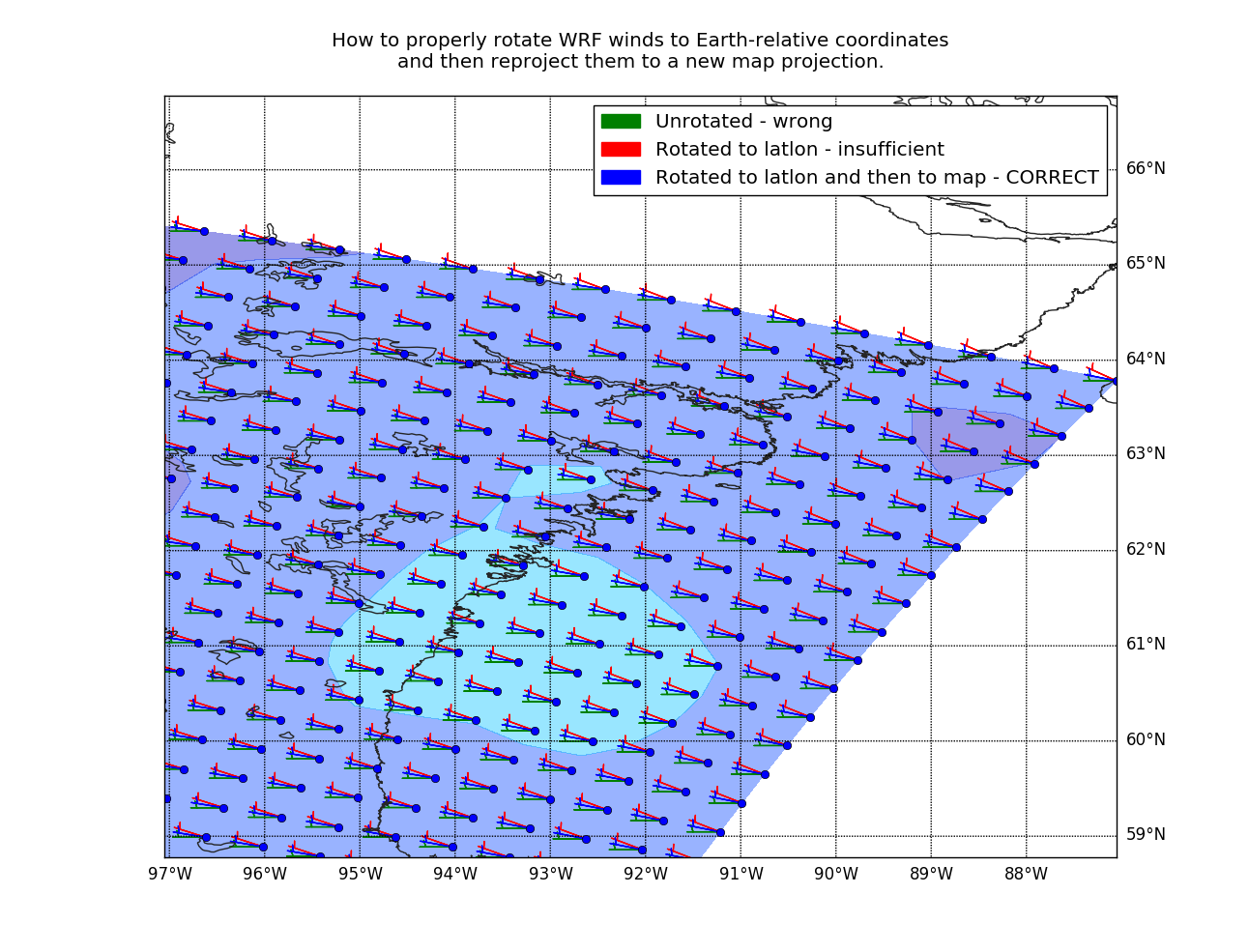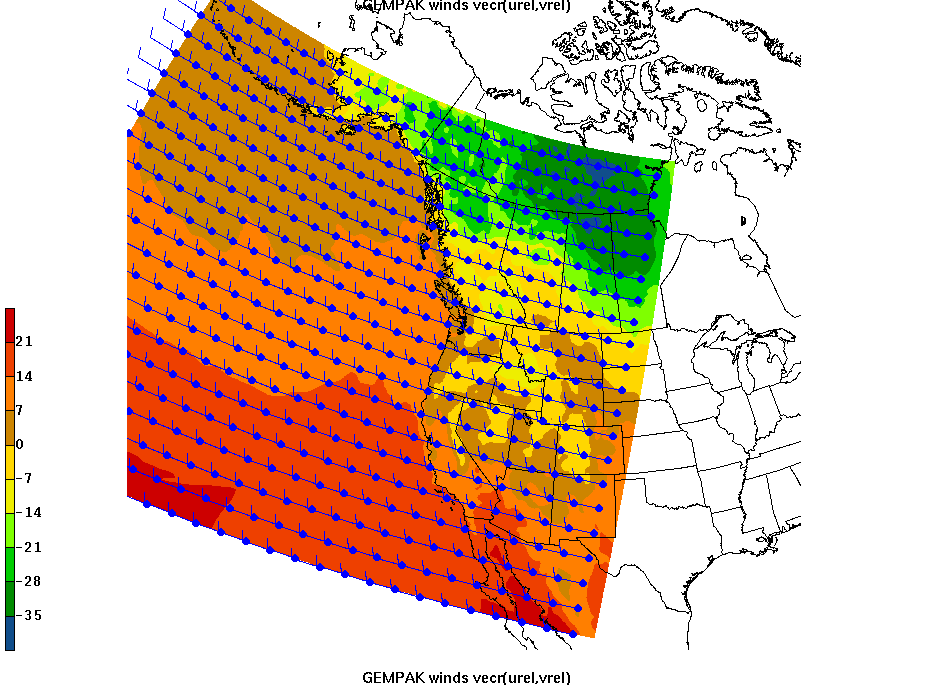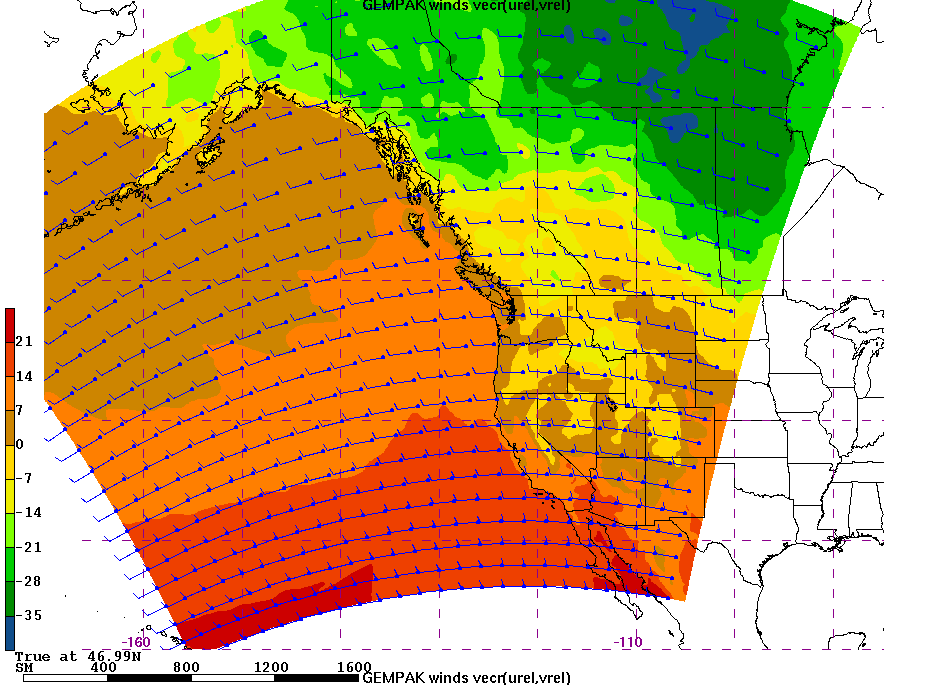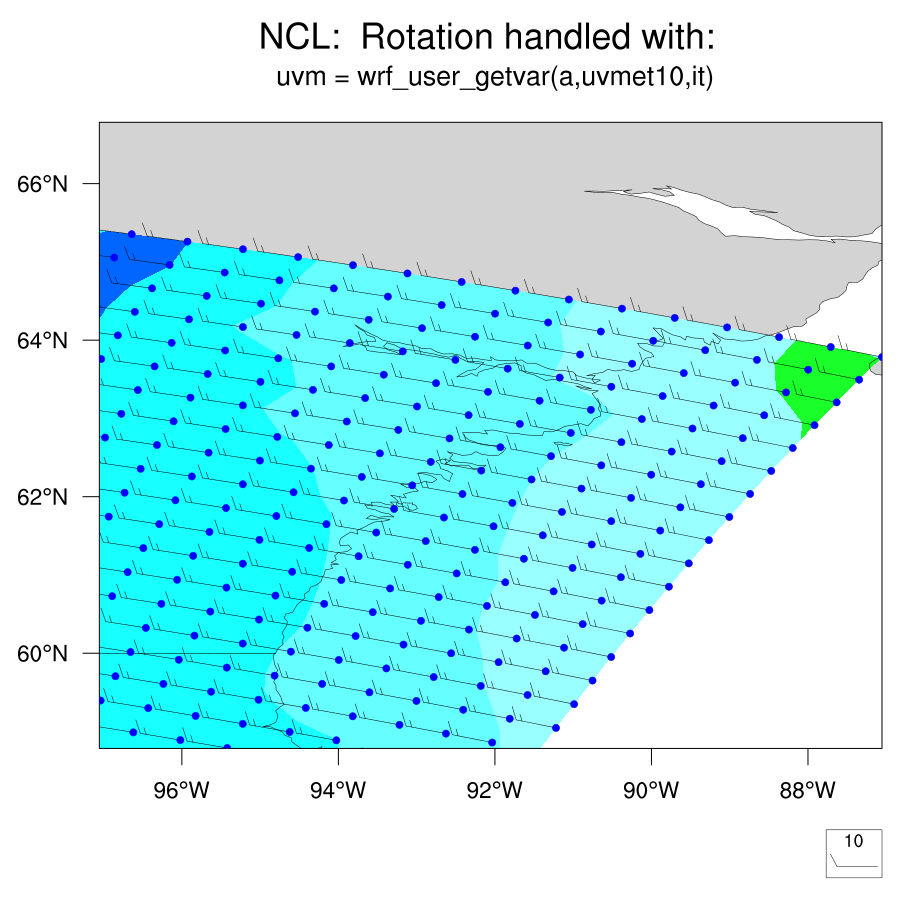# How to Properly Rotate WRF Winds to Earth-Relative CoordinatesUsing Python, GEMPAK, and NCL

by David Ovens, Department of Atmospheric Sciences, University of Washington

## Background

WRF is most often used as a regional model, using a Lambert Conformal Conic, Polar Stereographic, or Mercator projection. In all projections, WRF outputs the U and V components of the wind relative to the model grid, not relative to earth coordinates (where U would be west-east and V would be north-south). In the case of a Mercator projection, the model grid does align with earth coordinates and no rotation of the winds is needed. However, for the Lambert Conformal Conic and Polar Stereographic projections, a rotation is necessary whenever one wants to reproject the output or to calculate wind direction.

In my work, I have used a number of tools to rotate WRF winds to earth coordinates. The purpose of this page is to summarize and illustrate the problems, correct some formulas, and give some examples to help future users of WRF find a solution that works for them. I will illustrate solutions using NCL (the NCARGraphics Command Language), Python (using Basemap from matplotlib), GEMPAK, and read_wrf_nc (a WRF utility).

## Python Illustration

In this figure below I have attempted to illustrate the problem by modifying a WRF output file's U10 and V10 fields. The V10 field is set to 0 and the U10 field is simply 7 times the value of XLAND. The nco command used to generate these fields is:
`ncap2 -O -s 'V10=V10*0.' -s 'U10=XLAND*7.' -s 'V=V*0.' -s 'U=U*0.+10.' wrfout_d01_2016-03-02_15:00:00 wrfout_d1.uvrewrite.nc  `
The blue wind barbs are correctly rotated -- they line up with the model's grid points. They are produced by first finding the correct earth-relative components of the wind using the COSALPHA and SINALPHA fields from the WRF output:
```  Uearth = U*cosalpha - V*sinalpha
Vearth = V*cosalpha + U*sinalpha

NOTE: this is the correct version of these equations. Some sources on the Web incorrectly reverse the signs on the sinalpha terms.
```
Then, those earth-relative components are used along with the rotate_vector() function of matplotlib's basemap to get the new coordinates on the map's x/y grid.
```  Urot, Vrot = map.rotate_vector(Uearth,Vearth,lons,lats)
```
map.barbs() can then plot the winds correctly based on whatever projection you have chosen:
```  map.barbs(x, y, Urot, Vrot, color='blue')
```
The green wind barbs are the result of simply plotting U10 and V10. Note how they are parallel to the x axis of the plot.
The red wind barbs are the result of plotting the Uearth and Vearth winds without rotating them to the map's projection.Here is the python code that was used to generate this plot from this WRF output file:
```from netCDF4 import Dataset as NetCDFFile
from mpl_toolkits.basemap import Basemap
import matplotlib.pyplot as plt
import numpy as np
import sys
# allow specification of the wrfout file on the command line.
if (len(sys.argv) > 1):
ncfile = sys.argv
else:
ncfile = 'wrfout_d1.uvrewrite.nc'
print 'Using wrfout file: "','\b'+ncfile,'\b"' # use \b to delete space
nc     = NetCDFFile(ncfile, 'r')
#
# CEN_LAT and CEN_LON are specific to the nest and are not
# actually what you want.  If available, you should use
cenlat = nc.getncattr('CEN_LAT')
cenlon = nc.getncattr('CEN_LON')
cenlon = nc.getncattr('STAND_LON')
lat1   = nc.getncattr('TRUELAT1')
lat2   = nc.getncattr('TRUELAT2')
#
# get the actual longitudes, latitudes, and corners
lons = nc.variables['XLONG']
lats = nc.variables['XLAT']
lllon = lons[0,0]
lllat = lats[0,0]
urlon = lons[-1,-1]
urlat = lats[-1,-1]
#
# get the grid-relative wind components
ur = nc.variables['U10']
vr = nc.variables['V10']
#
# get the WRF local cosine and sines of the map rotation
cosalpha = nc.variables['COSALPHA']
sinalpha = nc.variables['SINALPHA']
#
# get the temperature field to plot a background color contour
t2m = nc.variables['T2']
#
# Make our map which is on a completely different projection.
# To illustrate the problems, go to the upper right corner of the domain,
# or lower right corner in the southern hemisphere, where the distortion
# is the greatest
if urlat >= 0:
map = Basemap(projection='cyl',llcrnrlat=urlat-5,urcrnrlat=urlat+3,
llcrnrlon=urlon-10,urcrnrlon=urlon,
resolution='h')
else:
map = Basemap(projection='cyl',llcrnrlat=lllat-5,urcrnrlat=lllat+3,
llcrnrlon=lllon,urcrnrlon=lllon+10,
resolution='h')
#
x, y = map(lons[:,:], lats[:,:])
map.contourf(x, y, t2m, alpha = 0.4)
#
# overlay wind barbs doing nothing to show the problem
map.barbs(x, y, ur, vr, color='green',label='Unrotated - wrong')
# rotate winds to earth-relative using the correct formulas
ue = ur * cosalpha - vr * sinalpha
ve = vr * cosalpha + ur * sinalpha
# overlay wind barbs using the earth-relative winds showing we're still wrong
map.barbs(x, y, ue, ve, color='red',label='Rotated to latlon - insufficient')
# now rotate our earth-relative winds to the x/y space of this map projection
urot, vrot = map.rotate_vector(ue,ve,lons,lats)
map.barbs(x, y, urot, vrot, color='blue',label='Rotated to latlon and then to map - CORRECT')
# finish up map drawing
parallels = np.arange(-85.,85,1.)
meridians = np.arange(-180.,180.,1.)
map.drawcoastlines(color = '0.15')
map.drawparallels(parallels,labels=[False,True,True,False])
map.drawmeridians(meridians,labels=[True,False,False,True])
# NOTE: for wind barb/vector plots, each component of the wind got its own
# legend entry, so this line
#   plt.legend()
# resulted in duplication.  The next 3 lines handle sifting the legend.
ax = plt.gca()
handles, labels = ax.get_legend_handles_labels()
legend = plt.legend([handles,handles,handles], [labels,labels,labels])
#
# plot the points of the grid to illustrate where the vectors should be pointing
map.plot(x,y,'bo')
plt.title('How to properly rotate WRF winds to Earth-relative coordinates\nand then reproject them to a new map projection.\n')
plt.show()
```
For the full U and V fields, they need to be unstaggered first. This can be done easily in python using this:
``` U = nc.variables['U']
V = nc.variables['V']
# destagger ala NCL
u_unstaggered = 0.5 * (U[:,:,:-1] + U[:,:,1:])
v_unstaggered = 0.5 * (V[:,:-1,:] + V[:,1:,:])
```

## GEMPAK Illustration

In GEMPAK, pass the U and V components into GEMPAK as UREL and VREL and then you can use GEMPAK's built-in VECR() function to tell programs that the winds are grid relative. The figures below plot winds with
```    gvect = vecr(urel,vrel)
or
gdpfun = vecr(urel,vrel)
```
NOTE: I was unable to get the proper winds setting PROJ = CED. However, setting PROJ to STR, LCC, MER all work fine.Here are the settings used to produce this last figure from gdplot2 using this simple10.gem file:

``` GDFILE   = simple10.gem
GDATTIM  = first
GLEVEL   = 10
GVCORD   = hagl
PANEL    = 0
SKIP     = 0/5
SCALE    = 0
GDPFUN   = tmpc@2 !vecr(ufix,vfix)
TYPE     = f ! BM
CONTUR   = 3
CINT     = 2
LINE     = 3/-3/3/2    ! 17/-3/2/2
FINT     = 0
FLINE    = 24-8
HILO     =
HLSYM    =
CLRBAR   = 32
WIND     = bm4/0.7
REFVEC   = 10
TITLE    = 32//GEMPAK winds vecr(urel,vrel)
TEXT     = 1/22/hw
CLEAR    = YES
GAREA    = 20;-170;65;-85
IJSKIP   =
PROJ     = mer
MAP      = 32
MSCALE   = 32;32
LATLON   = 30/2//5;5
DEVICE   = XW
STNPLT   =
SATFIL   =
IMCBAR   =
LUTFIL   =
STREAM   =
POSN     = 0
COLORS   = 1
MARKER   = 4/17/0.5
GRDLBL   = 0
FILTER   = no
```

## NCL Illustration

In NCL (the NCARGraphics Command Language) WRF wind rotation is made extremely easy by the use of the uvmet and uvmet10 fields in wrf_user_getvar:
```    wrf_user_getvar(filehandle,"uvmet",time)
wrf_user_getvar(filehandle,"uvmet10",time)
```
The figure below uses this function.Here is the code wrf_wind_reproj.ncl that produced that figure.
```;----------------------------------------------------------------------
; wrf_wind_reproj.ncl
;----------------------------------------------------------------------
; Concepts illustrated:
;   - using uvmet to rotate WRF grid-relative winds to earth-relative
;     and confirming this by reprojecting WRF output.
;   - Using gsn_csm_contour_map to plot WRF-ARW data
;   - Drawing a WRF lat/lon grid using gsn_coordinates
;----------------------------------------------------------------------
; This example is similar to wrf_gsn_3.ncl, except it zooms in further
; on the map and draws the WRF grid as points.
; Contributed March 2016 by David Ovens at
; http://www.atmos.washington.edu/~ovens/wrfwinds.html
;----------------------------------------------------------------------;

begin
;---Open WRF output file.
if (.not. isvar("f")) then      ; is f on command line?
f = "./wrfout_d1.uvrewrite.nc";
end if
a = addfile(f,"r")   ; Open a file

;---Read terrain height and lat/lon off file.
it        = 0     ; first time step
lats      = wrf_user_getvar(a,"XLAT",it)   ; latitude/longitude
lons      = wrf_user_getvar(a,"XLONG",it)  ; latitude/longitude

if(isatt(a,"STAND_LON")) then
cenlon = a@STAND_LON
else
cenlon = a@CEN_LON
end if
print("cenlon = "+cenlon)

u  = wrf_user_getvar(a,"U10",it)        ; u10 already on mass points
v  = wrf_user_getvar(a,"V10",it)        ; v10 already on mass points
t2m  = wrf_user_getvar(a,"T2",it)       ; temp at 2 meters in kelvin
dims = dimsizes(t2m)                     ;
print("dims = "+dims)
t2m  = t2m - 273.15                     ; now in celsius
nd = dimsizes(dims);
dimU = dims(nd-1) ;
dimV = dims(nd-2) ;
print("dimU = "+dimU+" dimV = "+dimV)

lllat = lats(0,0) ;
lllon = lons(0,0) ;
urlat = lats(dimV-1,dimU-1) ;
urlon = lons(dimV-1,dimU-1) ;
print("ll latlon ="+lllat+lllon+" ur latlon ="+urlat+urlon)

; NOTE: a lot of people assume that cen_lon is correct, but truly
; you want to use STAND_LON.  This is because you can set your
; longitude that goes exactly vertical in your WRF domain to be off
; the center.  STAND_LON is what you want.
;
; no need to do this:
;  uvm = wrf_uvmet(u,v,hgt@lat2d,hgt@lon2d,-121.,0.716)
; NCL can do the transformation internally:
uvm = wrf_user_getvar(a,"uvmet10",it) ; get it
; uc and vc calculated the long way:
;  cosalpha  = wrf_user_getvar(a,"COSALPHA",it)        ;
;  sinalpha  = wrf_user_getvar(a,"SINALPHA",it)        ;
;  uc = 1.94386 * (u*cosalpha - v*sinalpha)
;  vc = 1.94386 * (v*cosalpha + u*sinalpha)
; are identical to u,v calculated the easy way:
u = uvm(0,:,:)*1.94386     ; kts
v = uvm(1,:,:)*1.94386     ; kts
u@units = "kts"
v@units = "kts"
t2m@lat2d = lats           ;  required for plotting
t2m@lon2d = lons;          ;  required for plotting
t2m@description = "2m Temperature"
t2m@units = "C"
u@lat2d = lats           ;  required for plotting
u@lon2d = lons;          ;  required for plotting
v@lat2d = lats           ;  required for plotting
v@lon2d = lons;          ;  required for plotting
;  uc@lat2d = lats           ;  required for plotting
;  uc@lon2d = lons;          ;  required for plotting
;  vc@lat2d = lats           ;  required for plotting
;  vc@lon2d = lons;          ;  required for plotting

wks = gsn_open_wks("png","wrf_gsn")

;---Set some basic plot options
res               = True

res@gsnMaximize   = True   ; maximize plot in frame
res@gsnDraw       = False  ; don't draw
res@gsnFrame      = False  ; don't advance frame
res@gsnMaximize   = True   ; use full page
res@cnInfoLabelOn = False  ; turn off cn info label

res@mpProjection  = "CylindricalEquidistant"    ; The default

; Usually, when data is placed onto a map, it is TRANSFORMED to the
; specified projection. Since this model is already on a native lambert
; conformal grid, we want to turn OFF the tranformation.
;
res@tfDoNDCOverlay           = False

;---Zoom in on plot
res@mpMinLatF     = urlat-5
res@mpMaxLatF     = urlat+3.
res@mpMinLonF     = urlon-10
res@mpMaxLonF     = urlon

res@gsnAddCyclic             = False            ; regional data
res@vcRefMagnitudeF          = 10.0             ; define vector ref mag
res@vcRefLengthF             = 0.04            ; define length of vec ref
res@vcGlyphStyle             = "WindBarb"       ; WindBarb or CurlyVector
res@vcPositionMode           = "ArrowHead"      ; stick tail at value
res@vcMinDistanceF           = 0.001
res@gsnScalarContour        = True
res@cnFillOn                = True
res@cnFillDrawOrder         = "Draw"
res@cnFillPalette           = "gui_default"     ; set color map
res@cnLinesOn               = False             ; turn off contour lines
res@cnLineLabelsOn          = False             ; turn off contour lines

res@pmTickMarkDisplayMode = "Always"   ; nicer tickmarks

res@mpDataBaseVersion     = "MediumRes"       ; better and more map outlines
res@mpDataSetName         = "Earth..4"
res@mpOutlineBoundarySets = "AllBoundaries"
res@mpOutlineOn           = True
res@lbLabelBarOn          = False
res@lbTitleOn             = False

res@gsnCenterString          = "NCL:  Rotation handled with: ~C~ ~Z75~ uvm = wrf_user_getvar(a,uvmet10,it)"
res@gsnLeftString          = " ";
res@gsnRightString          = " ";
plot = gsn_csm_vector_scalar_map(wks,uc,vc,t2m,res)

mkres                 = True
mkres@gsMarkerSizeF  = 0.008
mkres@gsMarkerColor  = "blue"
gsn_coordinates(wks,plot,hgt,mkres)

end
```